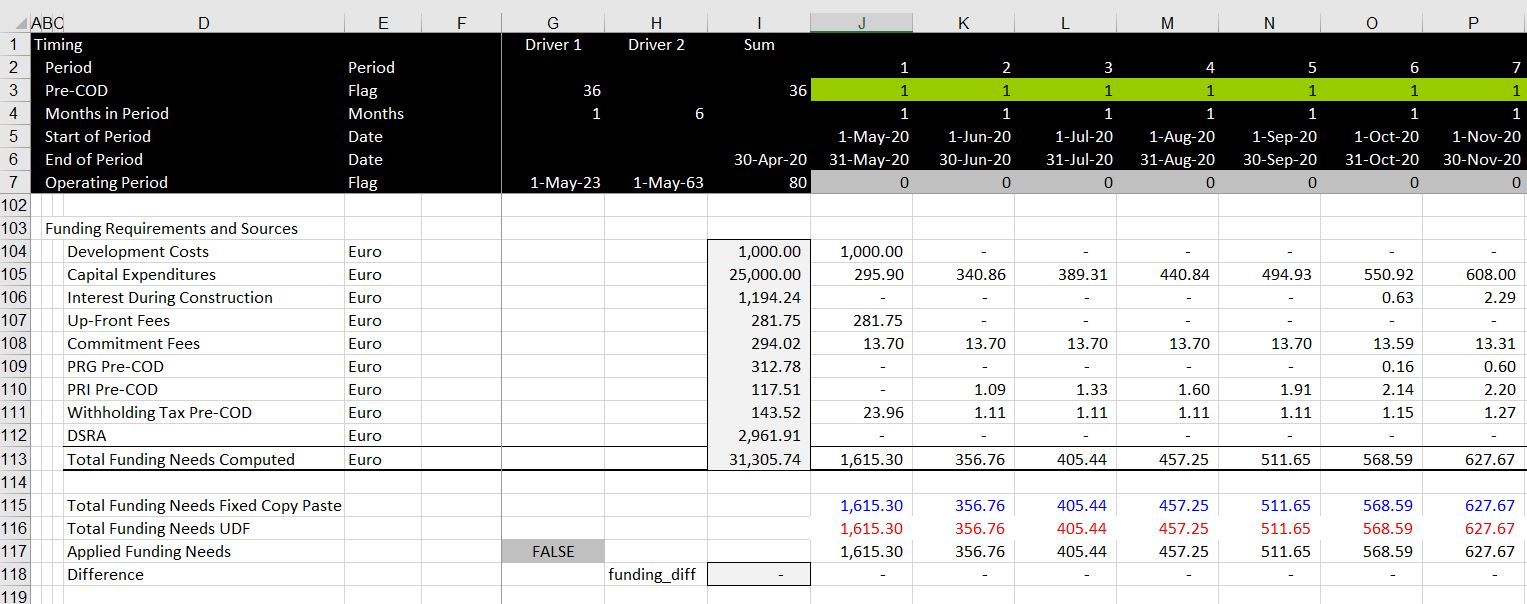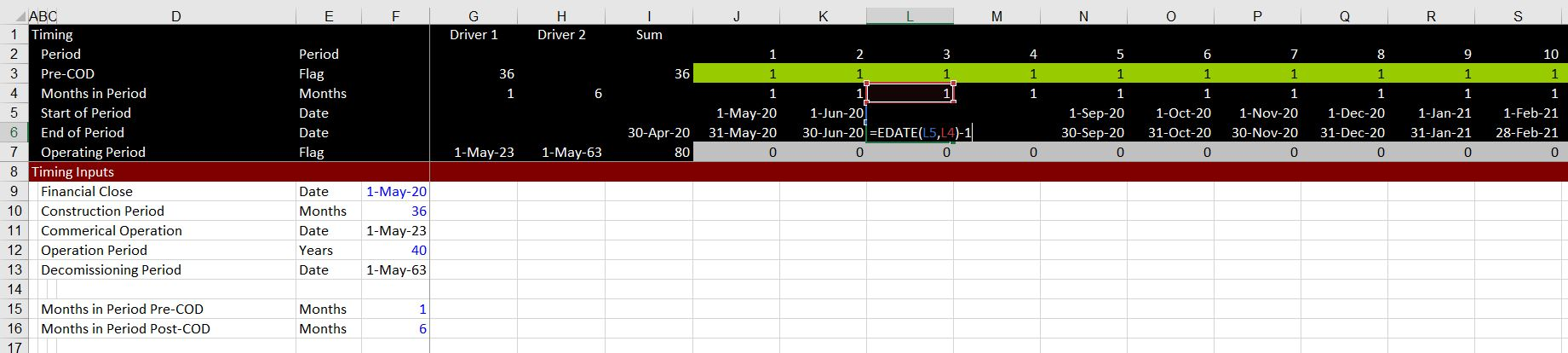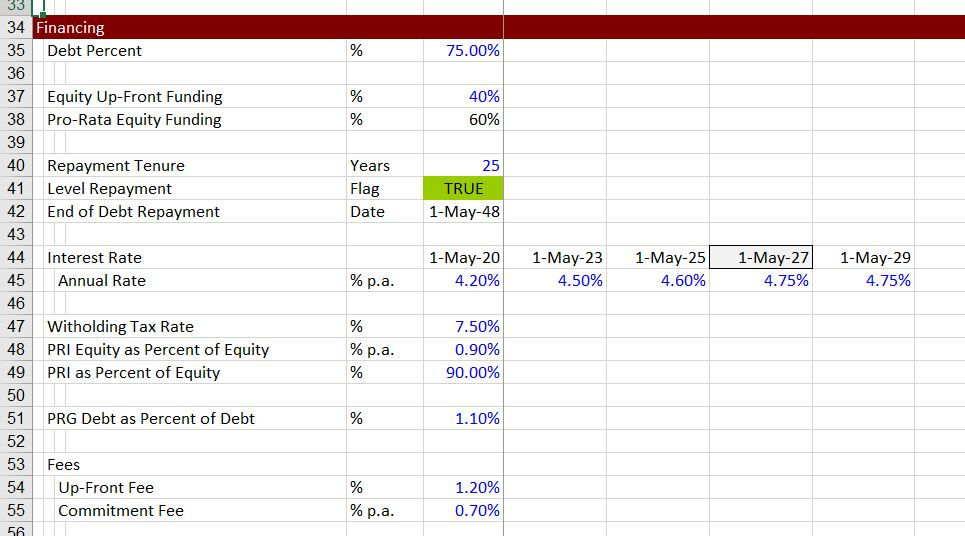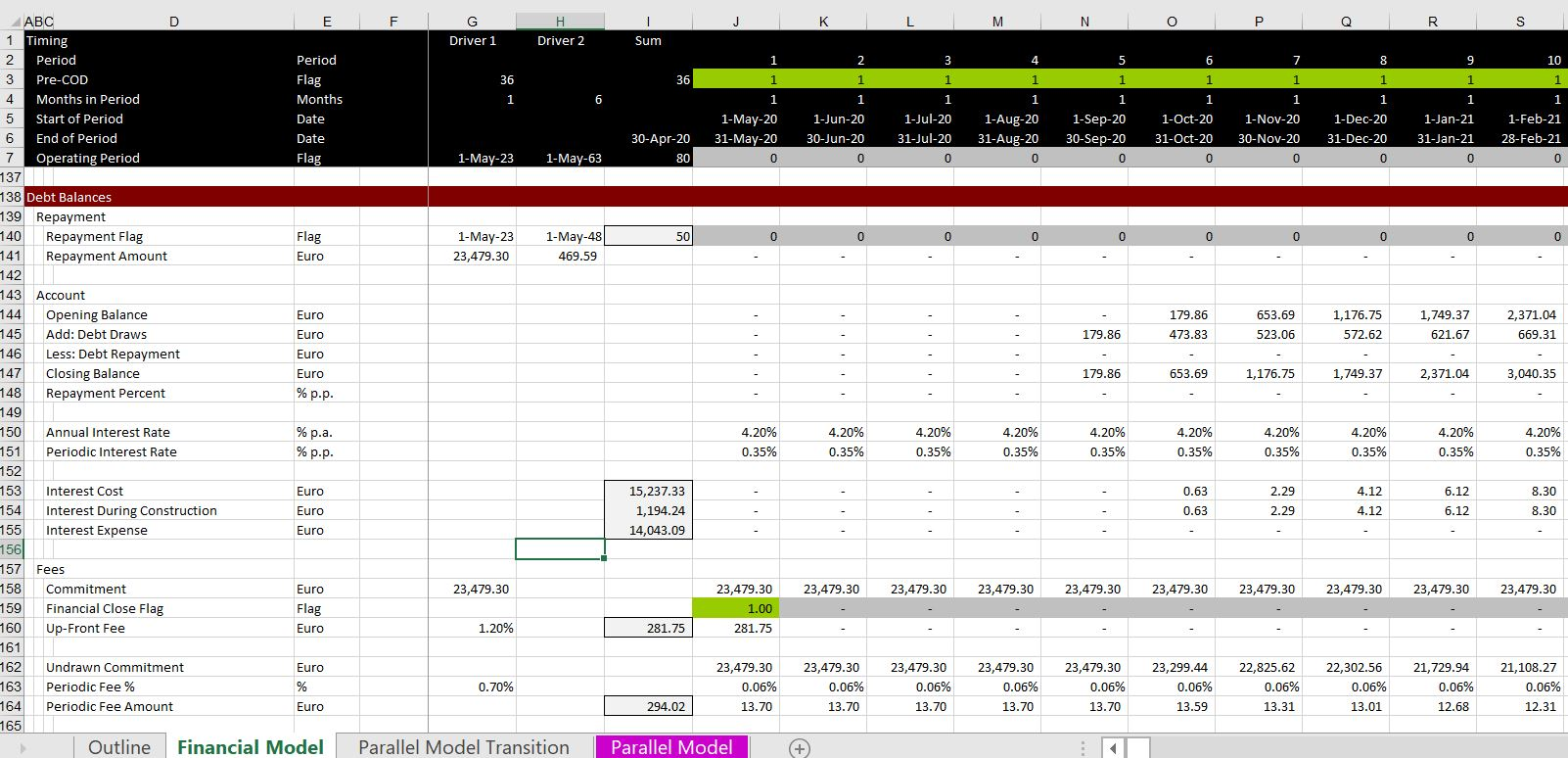# Implementing the UDF/Parallel Model Pre-COD

This webpage demonstrates how you can use the UDF/Parallel model to resolve circular references in a project finance model for interest, fees, withholding taxes, political risk insurance, ECA debt, shareholder debt, VAT debt and a DSRA account.  I first demonstrate how to use a classic copy and paste approach to circular references in project finance models. Then I illustrate how you can add the UDF approach to make your model more flexible and efficient allowing you to make better presentations.  The initial part of the webpage works through a single debt issue with PRG, PRI, withholding taxes and a DSRA account.  After this case with a single debt issue, more complex issues are addressed including how to model export credit agency debt (ECA), shareholder debt, a VAT facility along with political risk insurance. I have included a template model that allows you to add the UDF technique to your model in an without much pain at all and I hope I even illustrate how you can make changes to the code if you have minor changes to make.

I have included a few files in this section that allow you to see how the UDF/parallel model works.  The first is a template file that contains the UDF and can be added to the back-end of your model (to be more precise, you should add your model to the front-end of the UDF template).  The second file is an exercise file where you can create your own model in a manner that allows you to incorporate the UDF technique (if your model is a complete piece of crap with twenty different time lines and separate pages for construction financing and debt repayment, incorporating the UDF will be a bit more difficult).  The third file is the completed exercise file that you can use to try to add the UDF model yourself.  Once you have tried the exercise, you will hopefully be comfortable in adding this to your model. The fourth model includes VAT, shareholder debt, ECA debt and multiple debt issues.  This file illustrates some modelling techniques as well as how to incorporate the UDF/Parallel model with multiple different debt issues.

## Summary of Key Points in Using the UDF

At this point in the world with model auditors, large clumsy models tens of thousands of rows and incomprehensible presentation, using anything technique other than copy and paste macros to resolve circular references is controversial. In particular, when using the UDF technique, you may be quizzed on whether you understand every equation and why the technique is not a black box that is untransparent and impossible to verify. To resolve this issue I suggest that you show the UDF results right next to the model results from a copy and paste routine.  This is illustrated in the screenshot below.  I have created a little button and you can use either the copy and paste method or the UDF/Parallel model method.  For Pre-COD, this is pretty easy to do.  You can present a little analysis of a summary sources and uses of funds along with a copy and paste along with the UDF for the total funding requirements.  This manner of presenting the summaries for both the copy/paste and the UDF together is illustrated in the two screenshots below.  The first screenshot illustrates how you can make a summary sources and uses to demonstrate resolution of the funding circular references.  Without a DSRA account, this can resolve most of the circular references.  With a DSRA account there will often be a circular reference driven by the total funding needs.  This can be resolved with a copy and paste macro for the funding needs line.## Jae First Exercise with One Debt Issue — Review of the Financial Model and Reason for Circular References

My friend Jae from Korea has asked me for exercises which I think is a very good idea.  Incredibly, he has managed to write code for the UDF himself to resolve the circular references associated with model funding in project finance models. In this exercise I am interested to see how difficult it is to implement the UDF technique and to demonstrate that it can be implemented in a straightforward manner. In order to understand the UDF and the parallel model concept, I suggest that Jae tries to add the UDF code to an existing model with a copy and paste process. To do this you can open two of the excel files above — the second and the third file.  You can combine the UDF template that is associated with the third button above with the UDF template that is in the second button.  Hopefully this exercise with have you become more familiar with the UDF/parallel process and you will see how to reconcile the parallel models.

Along with the exercise where you attach the UDF template to your project finance model, you may rather work through building the project finance model.  By working with the model you can see some of the key inputs necessary for applying the UDF/Parallel Model such as a consistent time line, a continual interest rate time series, inputs that must be transferred and so forth.  I have put a video at the bottom of this section that works through the model on a step by step basis. The first screenshot below illustrates the development of a consistent time line that includes both the pre-COD and post-COD period.  To do this you can create a variable shown in the screenshot that has the months in the period dependent on the number of pre-COD periods.

Working through this model is very similar to the financing exercise in the A-Z project modelling section and I am sorry for the repetition. In each of the cases you can watch the video and work through the equations to build a model in a reasonable amount of time.After working through the capital expenditures and the EBITDA to develop pre-tax and after-tax project IRR, you can compute the summary sources and uses of funds shown above.  In the UDF, you need the total capital expenditure other than all of the financing cost items such as IDC, fees, VAT interest and so forth as well as the EBITDA.  The summary sources and uses may not be typical in a project finance model.  But as I already wrote above, it can be very good as a method for comparing the copy and paste method with the UDF/Parallel Model method.

In developing the model I use a method developed by Hedieh where you can input different amounts of equity investment up-front.  The equity up-front percentage can be zero in which case the financing would be pro-rata; or it can be 100%, in which case the financing would be equity-front.  This would cause a circular reference problem and result in a copy and paste macro in your traditional model.  The manner in which the up-front equity percent is applied is illustrated on the two screenshots below; first with the inputs and then with the calculations.  The input page below demonstrates that the equity up-front is 40% leaving the pro-rate to be 60%.The screenshot below which is too small outlines the calculations.  The total funding needs, like the total sources and uses have a circular reference problem.  The applied total funding needs is used to determine how much funding comes from up-front funding.  This could be 100% of the equity funding from the summary sources and uses if all equity were up-front or it could be less or it could be zero if there no up-front commitment.  Once the up-front equity is determined, the remainder comes from pro-rata.Once you have finished this part of the analysis, you can finish up the debt balance, the commitment fees, the up-front fees, the IDC, the political risk insurance, the withholding tax and the DSRA as shown illustrated in the screenshot below.  The circular reference comes about because these items are necessary to find the debt size, but the debt size drives the amount of these items.  Similarly, the circular reference is illustrated by the fact that you have to go down instead of going up to find items in equations of the model.After the debt balance and the rest of the fees, you can compute the political risk insurance that can be a function of the debt and equity balance.  Then you compute the withholding tax that is a function of the interest and fees.  At the end, you compute the DSRA account which is a function of the amount of debt service outstanding. I think that’s enough of the model set-up description.  Now lets go to the resolution of the circular reference.  I first discuss a bit of review of the copy and paste method and then move to how you can practically apply the UDF/Parallel model method.  The video at the bottom of the next section shows how to set-up this model.

## Resolution of Circular Reference with Copy and Paste Macros

I must tell you that in this case with only one debt issue and no debt sizing analysis and no sculpting analysis the copy and paste method is not that bad.  People who spend their lives working on models can very quickly make a copy and paste routine and if the model is set-up reasonably well, it is pretty clear where the copy and paste macros should go.  As shown above, the circular references come from the total debt balances and the total funding needs.  These items together with the CFADS if you have sculpting are the classic items that should be the focus of the circular reference.  The ease of making one of these macros is the reason that the copy and paste method has become common in models and is something that modellers are very proud of.

To create the copy and paste macro, you should remember that you copy from computed formulas to  fixed amounts in different cells.  I have repeated the sources and uses summary on the screenshot below and I have shown the range names associated with the computed and the fixed cells (you get this by pressing F3).  To make the copy and paste routine, you start recording and then copy and paste special from the computed to the fixed.  After that, you go to your macro and put in range names and a loop.  It is very easy once you get used to it.  Note that it can be a good idea to use the F3 short-cut to list the names.  You can then even import the names into your macro to avoid spelling mistakes.A similar copy and paste is used for the total funding needs where an entire array must be copied and pasted. The process is very similar and you can combine both copy and paste routines in the same macro.  In this case, shown in the screenshot below, you should make a range name for the entire row of numbers.  The place to copy and paste is demonstrated in the screenshot below.  Note that there is also a difference in the totals that is shown.  The macro will continue to copy and paste until this difference is zero.The code for the copy and paste macros are shown below.  Note that the differences in range names are summed and that a loop is put around the copy and paste.

```Sub copy_paste()
'
' computed_uses
' pasted_uses

Do While Range("funding_diff") + Range("uses_difference") <> 0

Range("funding_pasted").Value = Range("funding_needs").Value
Range("pasted_uses").Value = Range("computed_uses").Value

Loop

End Sub

.
.

```

## Combining the UDF Template with Financial Model and Entering Data in the UDF Template

To implement the UDF, together with or instead of the copy and paste, you can first open the UDF template that is connected to the button above. You can use ALT, E, M and then put your model into the template — not the other way around. The screenshot below illustrates the transition page of the UDF where you link various inputs to your model.  Once you link the inputs, the UDF creates a project finance model with a sources and uses of funds, sculpting and a complete post-COD financial analysis.Once you link the various inputs from your model and be careful not to link any variable that depends on debt (such as repayment), you can put the UDF outputs next door to the copy and paste outputs. Now your model is completely independent of the copy and paste interruption.  It may not seem like a big deal, but it opens up a large host of things you can do including dynamic debt sizing, flexible presentations, more speed, evaluation of different cash flows and their effect on cash flow as well as many other things.  In the next sections I demonstrate how the UDF/Parallel model works with multiple debt issues, shareholder debt, VAT debt facilities, an Equity Bridge Loan and application of a DSRA with and a letter of credit rather than a funded DSRA.

The first excerpt below demonstrates how to set-up a UDF where the output will go to more than one cell and be an array function. To do this, you begin by defining the function as a Variant as shown in the function definition below.  Then I define an output array.  The dimensions of the array are the row first and the column second.  The array dimensions just have to be large enough to cover the total number of rows and columns that will be created by the function.  As shown in the example, some sort of definition must be made of the output array.  Finally, the name of the function is assigned to output array.  The Option base 1 is defined at the very top of the page. When you use Option Base 1 the first row will be the item of the array with the number 1 rather than the number 0.

```Option Base 1

Function mult_sculpt() As Variant

Dim output(10, 100) As Single

' PUT IN THE CODE AND DEFINE OUTPUT

output(1, 1) = debt_irr
output(2, 1) = debt_balance

mult_sculpt = output

End Function```
```.
.```

### Work Backwards

Once you have created the structure of the UDF program, begin at the end and start working backwards. In this case, the end is computing the debt IRR.  The debt IRR is in turn computed from the debt cash flow which must be defined. Note that when you write the code, you can generally use excel functions with the WORKSHEETFUNCTION statement.  After you start at the end, keep working backwards until you have defined all the equations that are needed.

```.

Option Base 1

Function mult_sculpt() As Variant

Dim output(10, 100) As Single
Dim debt_cf(100) As Single

' Keep Going Backwards

debt_cf(i) = -debt_balance

For i = 2 To 40
For k = 1 To 3
debt_cf(i) = debt_cf(i) + debt_service(i, k)
Next k
Next i

debt_irr = WorksheetFunction.IRR(debt_cf)

output(1, 1) = debt_irr
output(2, 1) = debt_balance

mult_sculpt = output

End Function

.

```

### Continue to Work Backwards and Define Required Inputs

The next insert illustrates how to keep working backwards and compute the debt service for the different issues. You can make a loop that goes around the different debt issues and make the different calculation for the capture debt issue and the other debt issues.  In the calculation for the captured debt issue, the CFADS and the target DSCR are necessary.  These are inputs that will have to be read into the function.  For the non-captured debt, the sum of the debt service is necessary.
.

```Option Base 1

Dim output(10, 100) As Single
Dim debt_cf(100), debt_balance_issue(10), debt_cf(10, 100), cfads(100), llcr(10), non_capture_ds(100) As Single

' Keep Going Backwards

capture_k = 2

For k = 1 To 3
If k <> capture_k Then
For i = 1 To 40
debt_service(k, i) = cfads(i) / llcr(k)
non_capture_ds(i) = non_capture_ds(i) + debt_service(k, i)
Next i
End If
Next k

For k = 1 To 3
If k = capture_k Then
For i = 1 To 40
debt_service(k, i) = cfads(i) / target_dscr - non_capture_ds(i)
Next i
End If
Next k

debt_cf(i) = -debt_balance

For i = 2 To 40
For k = 1 To 3
debt_cf(i) = debt_cf(i) + debt_service(k, i)
Next k
Next i

debt_irr = WorksheetFunction.IRR(debt_cf)

output(1, 1) = debt_irr
output(2, 1) = debt_balance

For i = 1 To 40
output(3, i) = debt_cf(i)
Next i

mult_sculpt = output

End Function```

.

.

### Create and Iteration Loop for Circular Reference

After you have worked through the equations you can make an iteration loop.  I am often lazy about this, but it is best to put in a loop that goes around until the circular reference is solved.  When I am lazy I just use a simple FOR NEXT loop.  But it is best to find one of the sources of circular reference. Then you can see when the difference between the current iteration and the last iteration for that variable declines to zero or almost zero.  To do this you better make sure that the very first iteration does not go to zero.

Further Information and Learning: Request Resource Library (Free), Details About Courses

.

```last_debt_balance = 999
debt_balance_difference = 999

Do While abs(debt_balance_difference) > .010

last_debt_balance = debt_balance

' All the stuff

debt_balance_difference = last_debt_balance - debt_balance

.```

.

### Final Circular Resolution

.

```.

Function mult_sculpt(cfads, target_dscr, target_pct1, tenure1, int_rate1, _
target_pct2, tenure2, int_rate2, target_pct3, tenure3, int_rate3) As Variant

Dim output(10, 100) As Single
Dim debt_cf(100), cfads1(100), debt_balance_issue(10), llcr(10), non_capture_ds(100) As Single
Dim debt_service(10, 100), debt_service_capture(100), target_pct(10), tenure(10), int_rate(10) As Single
Dim pv_cash_flow(10), cfads_issue(100), overall_debt_service(100) As Single

' Keep Going Backwards

capture_k = 2

tenure(1) = tenure1
tenure(2) = tenure2
tenure(3) = tenure3

max_tenure = WorksheetFunction.Max(tenure1, tenure2, tenure3)

target_pct(1) = target_pct1
target_pct(2) = target_pct2
target_pct(3) = target_pct3

int_rate(1) = int_rate1
int_rate(2) = int_rate2
int_rate(3) = int_rate3

last_debt_balance = 999
debt_balance_difference = 999
iter = 0
debt_irr = int_rate(1)

' Do While Abs(debt_balance_difference) < 0.01

For j = 1 To 15

iter = iter + 1

If iter > 20 Then Exit Function

last_debt_balance = debt_balance

' All the stuff

For i = 1 To 40
If target_dscr <> 0 And i <= max_tenure Then overall_debt_service(i) = cfads(i) / target_dscr
non_capture_ds(i) = 0
debt_cf(i) = 0
Next i

overall_debt_balance = WorksheetFunction.NPV(debt_irr, overall_debt_service)

For k = 1 To 3
If k <> capture_k Then
For i = 1 To 40

If i <= tenure(k) Then
End If
Next i

debt_balance_issue(k) = target_pct(k) * overall_debt_balance

llcr(k) = 1

If (debt_balance_issue(k) <> 0) Then llcr(k) = pv_cash_flow(k) / debt_balance_issue(k)

For i = 1 To 40
If i <= tenure(k) Then
debt_service(k, i) = 0
If (llcr(k) > 1) Then debt_service(k, i) = cfads(i) / llcr(k)
non_capture_ds(i) = non_capture_ds(i) + debt_service(k, i)
End If
Next i
End If
Next k

For k = 1 To 3
If k = capture_k Then
For i = 1 To 40
If i <= tenure(k) Then debt_service(k, i) = cfads(i) / target_dscr - non_capture_ds(i)
debt_service_capture(i) = debt_service(k, i)
Next i
debt_balance_issue(k) = WorksheetFunction.NPV(int_rate(k), debt_service_capture)
End If
Next k

debt_balance = 0
For k = 1 To 3
debt_balance = debt_balance + debt_balance_issue(k)
Next k

debt_cf(1) = -debt_balance

For i = 2 To 40
For k = 1 To 3
debt_cf(i) = debt_cf(i) + debt_service(k, i - 1)
Next k
Next i

debt_irr = WorksheetFunction.IRR(debt_cf, 0.01)

debt_balance_difference = last_debt_balance - debt_balance

' Loop

Next j

output_1:

output(1, 1) = debt_irr
output(2, 1) = debt_balance

For i = 1 To 30
output(3, i) = debt_cf(i)
Next i

For k = 1 To 3
output(4, k) = debt_balance_issue(k)
Next k
output(4, 4) = overall_debt_balance

For i = 1 To 40
Next i

For i = 1 To 40
output(6, i) = overall_debt_service(i)
Next i

For i = 1 To 40
output(7, i) = non_capture_ds(i)
Next i

For i = 1 To 40
output(8, i) = debt_service_capture(i)
Next i

For k = 1 To 3
output(9, k) = llcr(k)
Next k

For k = 1 To 3
output(9, k + 4) = pv_cash_flow(k)
Next k

mult_sculpt = output

End Function```

.

.